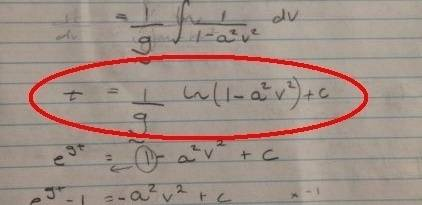# Difficult integral - Falling mass

## Homework Statement

Solve the differential equation, dt/dv= 1/g (1/1-a^2*v^2) where a = (k/mg)^1/2 to yield v= 1/a(1-e^-2agt/1+e^-2agt).

F=ma
Newton's 2nd Law
Integration Laws

## The Attempt at a Solution

See image. I think I'm getting messed up on the integration laws.

#### Attachments

•image.jpeg
37 KB · Views: 357

SteamKing
Staff Emeritus
Homework Helper

Solving differential equations and calculating integrals are by nature calculus problems, not pre-calculus.

Ray Vickson
Homework Helper
Dearly Missed

## Homework Statement

Solve the differential equation, dt/dv= 1/g (1/1-a^2*v^2) where a = (k/mg)^1/2 to yield v= 1/a(1-e^-2agt/1+e^-2agt).

F=ma
Newton's 2nd Law
Integration Laws

## The Attempt at a Solution

See image. I think I'm getting messed up on the integration laws.

$$\frac{dt}{dv} = \frac{1}{g} \left( \frac{1}{1} - a^2 v^2 \right) = \frac{1}{g} ( 1 - a^2 v^2)$$
(which is what you wrote) does not have the solution
$$v = \frac{1}{a} \left( 1 - e^{-2} \frac{agt}{1} + e^{-2} agt \right)$$
which is what you wrote. If these are not what you mean, you need to start using parentheses.

I did not look at the attached image; I look only at typed work, which is the preferred format in this Forum.

$$\frac{dt}{dv} = \frac{1}{g} \left( \frac{1}{1} - a^2 v^2 \right) = \frac{1}{g} ( 1 - a^2 v^2)$$
(which is what you wrote) does not have the solution
$$v = \frac{1}{a} \left( 1 - e^{-2} \frac{agt}{1} + e^{-2} agt \right)$$
which is what you wrote. If these are not what you mean, you need to start using parentheses.

I did not look at the attached image; I look only at typed work, which is the preferred format in this Forum.

Sorry for the misconception. I meant to write:
dt/dv = 1/g ((1)/(1-a^2v^2))
V = 1/a ((1-e^(-2agt))/(1+e^(-2agt)))

Hopefully this is clearer.

Samy_A
Homework Helper
Sorry for the misconception. I meant to write:
dt/dv = 1/g ((1)/(1-a^2v^2))
V = 1/a ((1-e^(-2agt))/(1+e^(-2agt)))

Hopefully this is clearer.
Try to use LaTeX (https://www.physicsforums.com/help/latexhelp/ )
Do you mean:
##\displaystyle \frac{dt}{dv}= \frac{1}{g}\frac{1}{1-a²v²}##
##\displaystyle v=\frac{1}{a}\frac{1-e^{-2agt}}{1+e^{-2agt}}##?

$\frac{dt} {dv} = \frac{1} {g} ( \frac{1} {1 - a^2 v^2} )$

$v = \frac{1} {a} ( \frac{1 - e^(-2agt)} {1 + e^(-2agt)} )$

The (-2agt) is to the power of e
e^(-2agt)
Sorry for the confusion guys!! :(

Try to use LaTeX (https://www.physicsforums.com/help/latexhelp/ )
Do you mean:
##\displaystyle \frac{dt}{dv}= \frac{1}{g}\frac{1}{1-a²v²}##
##\displaystyle v=\frac{1}{a}\frac{1-e^{-2agt}}{1+e^{-2agt}}##?

$\frac{dt} {dv} = \frac{1} {g} ( \frac{1} {1 - a^2 v^2} )$

$v = \frac{1} {a} ( \frac{1 - e^{-2agt}} {1 + e^{-2agt}} )$

This is what I mean to write.
Sorry for the confusion guys!! :(

•Samy_A
Samy_A
Homework Helper
Great!

Now back to the exercise:Something went wrong in the part I circled in red.
The derivative of ##\log (1-a²v²)## is ##\frac{-2av}{1-a²v²}##.

EDIT: To fix the error above: the derivative of ##\log (1-a²v²)## is ##\frac{-2a²v}{1-a²v²}##.

Last edited:
Great!

Now back to the exercise:

View attachment 98245

Something went wrong in the part I circled in red.
The derivative of ##\log (1-a²v²)## is ##\frac{-2av}{1-a²v²}##.

Yeh, I know there is probably an issue here in my work, but I wrote: ##\ln (1 - a^2 v^2)## when derived, this gives ##\frac{1}{1-a^2 v^2}## doesn't it?

Samy_A
Homework Helper
Yeh, I know there is probably an issue here in my work, but I wrote: ##\ln (1 - a^2 v^2)## when derived, this gives ##\frac{1}{1-a^2 v^2}## doesn't it?
No, it gives ##\frac{-2a²v}{1-a^2 v^2}## (I forgot the a² in my previous post).

You have to apply the chain rule.
If ##f(x)=\ln g(x)##, then ##f'(x)=\frac{g'(x)}{g(x)}##.

No, it gives ##\frac{-2a²v}{1-a^2 v^2}## (I forgot the a² in my previous post).

You have to apply the chain rule.
If ##f(x)=\ln g(x)##, then ##f'(x)=\frac{g'(x)}{g(x)}##.
Yeahh I'm really stuck on this one. I don't know how I can get my ##g'(x) = 1## whilst the bottom ##= 1 - a^2 v^2##??

Samy_A